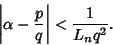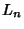## Lagrange Number (Rational Approximation)

Hurwitz's Irrational Number Theorem gives the best rational approximation possible for an arbitrary irrational numberasTheare called Lagrange numbers and get steadily larger for each bad'' set of irrational numbers which is excluded.Exclude1 none23Lagrange numbers are of the formwhereis a Markov Number. The Lagrange numbers form a Spectrum called the Lagrange Spectrum.

See also Hurwitz's Irrational Number Theorem, Liouville's Rational Approximation Theorem, Liouville-Roth Constant, Markov Number, Roth's Theorem, Spectrum Sequence, Thue-Siegel-Roth Theorem

References

Conway, J. H. and Guy, R. K. The Book of Numbers. New York: Springer-Verlag, pp. 187-189, 1996.